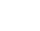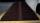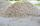# Pyramid Problems

#### Number of problems found: 116

• Distance of pointsA regular quadrilateral pyramid ABCDV is given, in which edge AB = a = 4 cm and height v = 8 cm. Let S be the center of the CV. Find the distance of points A and S.
• House roofThe roof of the house has the shape of a regular quadrangular pyramid with a base edge 17 m. How many m2 is needed to cover roof if roof pitch is 57° and we calculate 11% of waste, connections and overlapping of area roof?
• The Scout TentThe Scout Tent has a rectangular wooden underlay with dimensions of 220 cm and 150 cm. How much canvas is needed for a 170 cm high of pyramid roof?
• Secret treasureScouts have a tent in the shape of a regular quadrilateral pyramid with a side of the base 4 m and a height of 3 m. Determine the radius r (and height h) of the container so that they can hide the largest possible treasure.
• Digging a pitThe pit has the shape of a regular quadrilateral truncated pyramid. The edges of the bases are 14m and 10m long. The sidewalls form an angle of 135° with a smaller base. Determine how many m3 of soil were excavated when digging the pit?
• Roof coverAbove the pavilion with a square ground plan with a side length of a = 12 m is a pyramid-shaped roof with a height v = 4.5 m. Calculate how much m2 of sheet metal is needed to cover this roof if 5.5% of the sheet we must add for joints and waste.
• FlowerbedFlowerbed has the shape of a truncated pyramid, the bottom edge of the base a = 10 m, the upper base b = 9 m. Deviation angle between edge and the base is alpha = 45°. What volume is needed to make this flowerbed? How many plants can be planted if 1 m2 =
• PitThe pit has the shape of a truncated pyramid with a rectangular base and is 0.8 m deep. The pit's length and width are the top 3 × 1.5 m bottom 1 m × 0.5 m. To paint one square meter of the pit we use 0.6 l of green color. How many liters of paint are nee
• TetrahedronCalculate height and volume of a regular tetrahedron whose edge has a length 4 cm.
• Chocholate pyramidHow many chocolates are in the third shelf when at the 8th shelf are 41 chocolates in any other shelf is 7 chocolates more the previous shelf.
• ConeThe rotating cone volume is 9.42 cm3, with a height 10 cm. What angle is between the side of the cone and its base?
• Mystery of stereometrieTwo regular tetrahedrons have surfaces 88 cm2 and 198 cm2. In what ratio is their volumes? Write as a fraction and as a decimal rounded to 4 decimal places.
• Lamp coneCalculate the surface of a lamp shade shaped of a rotary truncated cone with base diameter 32 cm and 12 cm and height 24 cm.
• Gravel - coneThe mound of gravel has a regular circular cone shape with a height 3.3 meter and a base circumference of 18.85 meters. How many cubic meters of gravel is in a pile? Calculate the weight of gravel if its density is p = 640 kg / cubic m.
• Hexagon rotationA regular hexagon of side 6 cm is rotated through 60° along a line passing through its longest diagonal. What is the volume of the figure thus generated?
• Billiard ballsA layer of ivory billiard balls of radius 6.35 cm is in the form of a square. The balls are arranged so that each ball is tangent to every one adjacent to it. In the spaces between sets of 4 adjacent balls other balls rest, equal in size to the original.

Do you have an interesting mathematical word problem that you can't solve it? Submit a math problem, and we can try to solve it.

We will send a solution to your e-mail address. Solved examples are also published here. Please enter the e-mail correctly and check whether you don't have a full mailbox.

Please do not submit problems from current active competitions such as Mathematical Olympiad, correspondence seminars etc...